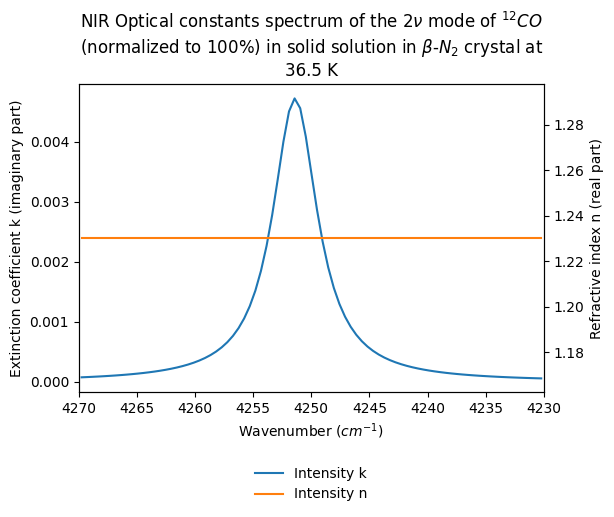Spectrum previewTitle
NIR Optical constants spectrum of the $2\nu$ mode of $^{12}CO$ (normalized to 100%) in solid solution in $\beta$-$N_2$ crystal at 36.5 K
Database
Experiment type
laboratory measurement
Spectrum type
optical constants
Version
#1 (2017-11-17 17:16:56 UTC+0000)
Instrument parameters
Instrument
Nicolet 800 – transmission Near-IR
Spectral observation mode
spectrum
Valid spectral range(s)
4230.0 - 4270.0 $cm-1$
Spatial observation mode
single spot
Sample preview
Name
CO in beta-N2 crystalline
Origin
laboratory
Texture
compact crystal
Thickness
1.0 $\pm$ 0.01 $mm$
Temperature
36.5 $\pm$ 0.3 $K$
Fluid
• $N_2$ , 99.55000000000001 $\pm$ 0.005%
• $CO$ , 0.44999999999999996 $\pm$ 0.005%
References
Data reference
Quirico, Eric; Schmitt, Bernard (1992): NIR Optical constants spectra of the $2\nu$ mode of $^{12}CO$ in solid solution in $N2$ crystal at differents temperatures. SSHADE/GhoSST (OSUG Data Center). Dataset/Spectral Data.